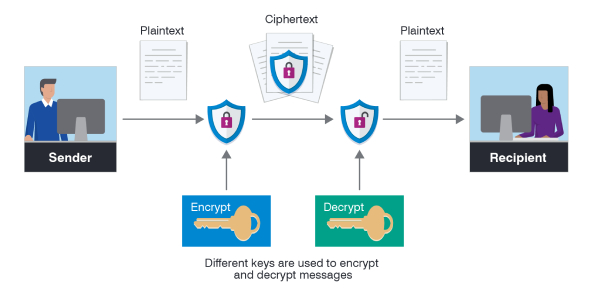# Encryption Algorithm Trivia Quiz!

32 Questions | Attempts: 260Settings.

• 1.
_____________________ defines what size of plain text should be encrypted in each step of algorithm.
• A.

Algorithm types

• B.

Stream cipher

• C.

Algorithm mode

• D.

Block cipher

• 2.
______________ is a technique of ensuring that the cipher text gives no clue about original plain text.
• A.

Diffusion

• B.

Confusion

• C.

• D.

Distraction

• 3.
_______________ is simply used to make each message unique.
• A.

Encryption

• B.

Decryption

• C.

Initial Vector

• D.

Initialization Vector

• 4.
Which of these is an algorithm mode?
• A.

Electronic cipher book

• B.

Cipher block chaining

• C.

Counter feedback mode

• D.

Output chaining mode

• 5.
DES has how many rounds?
• A.

8

• B.

16

• C.

32

• D.

64

• 6.
IP stands for what in DES?
• A.

Internet Protocol

• B.

Initial Protocol

• C.

Initial Permutation

• D.

None of these

• 7.
In the 16 rounds of DES , the first round is?
• A.

Key transformation

• B.

Expansion permutation

• C.

Key generation

• D.

S-box substitution

• 8.
IDEA stands for?
• A.

Integrated Data Encryption Algorithm

• B.

International Data Encryption Algorithm

• C.

Internation Data Encoding algorithm

• D.

None of these

• 9.
IDEA has ___ rounds.
• A.

8

• B.

16

• C.

32

• D.

64

• 10.
RC4 was invented in the year______
• A.

1985

• B.

1986

• C.

1987

• D.

1988

• 11.
Blowfish has was developed by?
• A.

Ron Rivest

• B.

Bruce Schneier

• C.

Brack Schneier

• D.

None of these

• 12.
In RSA, the first step is to accept two large prime numbers.
• A.

Q and N

• B.

P and Q

• C.

P and N

• D.

All of the above

• 13.
What defines the details of the cryptographic algorithm?
• A.

Algorithm types

• B.

Algorithm modes

• C.

Both

• D.

None

• 14.
____________ defines the encryption of one plain text at a time.
• A.

Plain text

• B.

Cipher text

• C.

Stream cipher

• D.

Block cipher

• 15.
What defines the encryption of one block of bit at a time?
• A.

Plain text

• B.

Cipher text

• C.

Stream cipher

• D.

Block cipher

• 16.
HMAC is a type of?
• A.

Message digest

• B.

Digital signature

• C.

Both

• D.

None

• 17.
MAC stands for?
• A.

Message Authentication Code

• B.

Message Authentication control

• C.

Message Authentication cipher

• D.

None

• 18.
Which is the most simplest mode in algorithm mode?
• A.

Cipher block chaining

• B.

Electronic code book

• C.

Cipher feedback mode

• D.

Counter mode

• E.

Output feedback mode

• 19.
Initial permutation divides the plain text into two halves of?
• A.

32 bits

• B.

64 bits

• C.

128 bits

• D.

It is not divided

• 20.
The last step of des is
• A.

Initial permutation

• B.

S box substitution

• C.

Final permutation

• D.

None of the above

• 21.
Which of the following is the objective  Of blowfish?
• A.

Fast

• B.

Compact

• C.

Simple

• D.

All of thes

• 22.
RSA is a________
• A.

Symmetric

• B.

Asymmetric

• C.

Both

• D.

None

• 23.
In rsa algorithm n is calculated as?
• A.

P + Q

• B.

P-Q

• C.

P*Q

• D.

None

• 24.
Select a public key E such that it should not be a factor of (P-1) and (Q-1)
• A.

True

• B.

False

• 25.
The blowfish algorithm executes the ____________ algorithm for subkey generation.
• A.

Blowfish

• B.

IDEA

• C.

Rijndael

• D.

RC4

## Related TopicsBack to top
×

Wait!
Here's an interesting quiz for you.